We all know that the shortest path between two points on a plane is a straight line. On the surface of a sphere, however, there are no straight lines. The shortest path between two points on the surface of a sphere is given by the arc of the great circle passing through the two points. A great circle is defined to be the intersection with a sphere of a plane containing the centre of the sphere. If the plane does not contain the centre of the sphere, its intersection with the sphere is known as a small circle. In more everyday language, if we take an apple, assume it is a sphere, and cut it in half, we slice through a great circle. If we make a mistake, miss the centre and hence cut the apple into two unequal parts, we will have sliced through a small circle. Figure 1 illustrates the concepts of great and small circles.

 figure 1: upper panel: examples of great circles. lower panel: examples of small circles. The black dot represents the centre of the sphere.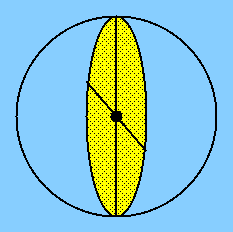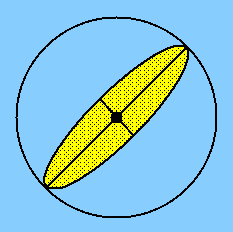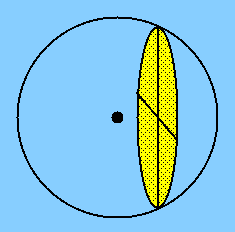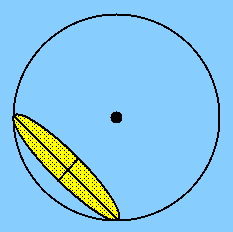Another concept we should all be familiar with from planar geometry is the triangle. If we wish to connect three points on a plane using the shortest possible route, we would draw straight lines and hence create a triangle. By analogy, if we wish to connect three points on the surface of a sphere using the shortest possible route, we would draw arcs of great circles and hence create a spherical triangle. To avoid ambiguities, a triangle drawn on the surface of a sphere is only a spherical triangle if it has all of the following properties:
• The three sides are all arcs of great circles.
• Any two sides are together greater than the third side.
• The sum of the three angles is greater than 180°.
• Each spherical angle is less than 180°.
Hence, in Figure 2, triangle PAB is not a spherical triangle (as the side AB is an arc of a small circle), but triangle PCD is a spherical triangle (as the side CD is an arc of a great circle). You can see that the above definition of a spherical triangle also rules out the "triangle" PCED as a spherical triangle, as the vertex angle P is greater than 180° and the sum of the sides PC and PD is less than CED.

 figure 2: Triangle PCD is a spherical triangle. Triangles PAB and PCED are not spherical triangles.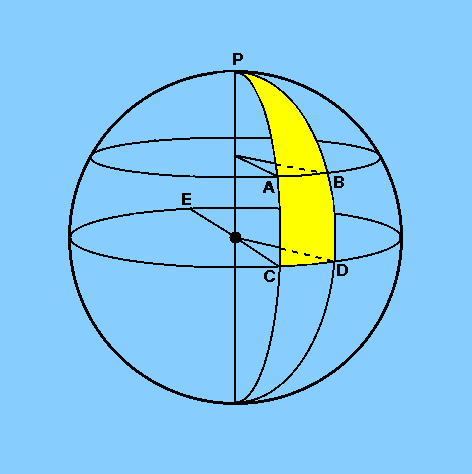It is important to realise that the lengths of the sides of a spherical triangle are expressed in angular measure. This follows from the fact that the length of an arc s which subtends an angleat the centre of a circle of radius r is given by

s = (/ 360) . 2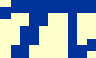r

whereis measured in degrees and s is given in whatever length units are used for r. Recalling that 2radians = 360°, this gives

s =r

whereis now measured in radians and s is still given in whatever length units are used for r. Given that spherical triangles are all made up of great circle arcs, and all great circle arcs on a sphere are of the same radius, it is convenient to take the radius of the sphere as unity and then write

s =which shows that the length of a side of a spherical triangle is equal to the angle (in radians) it subtends at the centre of the sphere. For example, if s is one-quarter of the circumference of a great circle, s is then/ 2 radians and there is no ambiguity if it is then expressed as 90°.